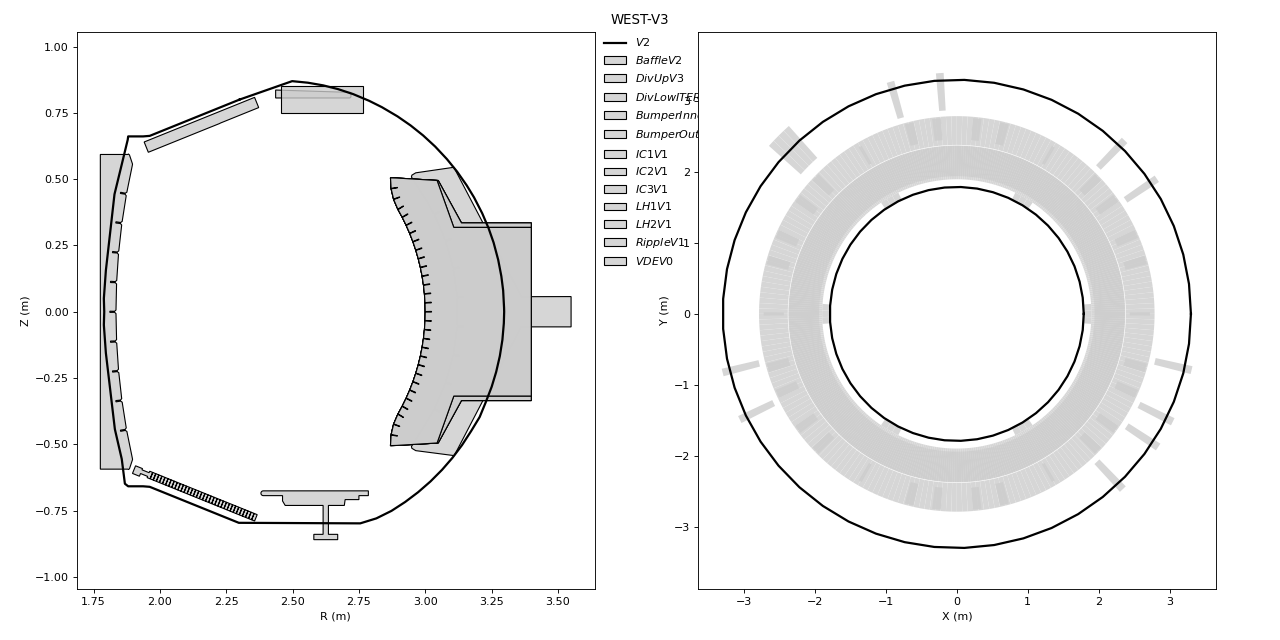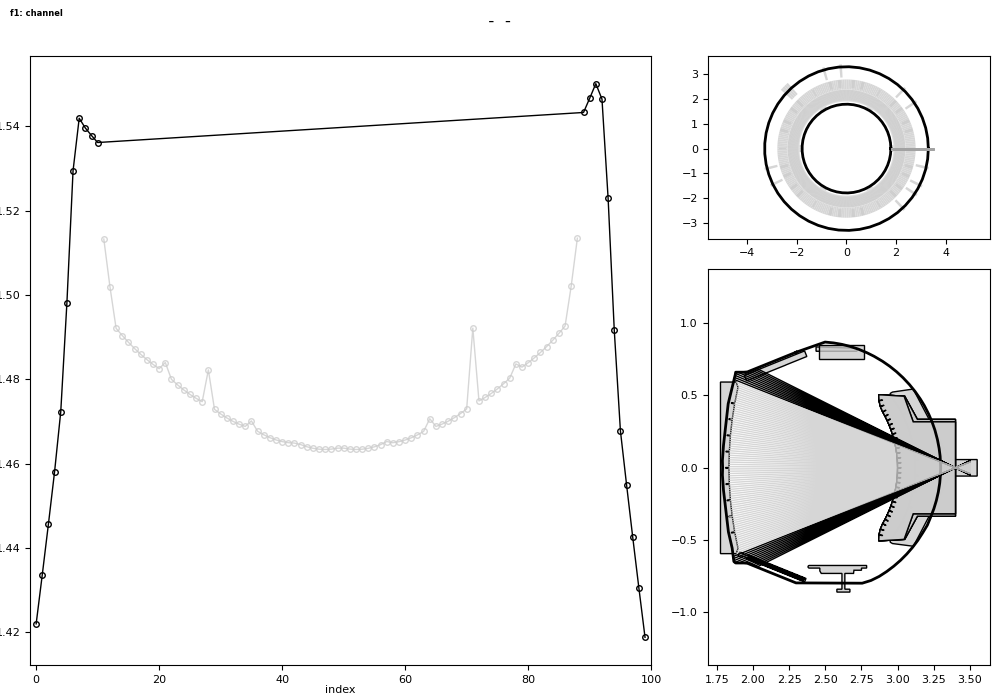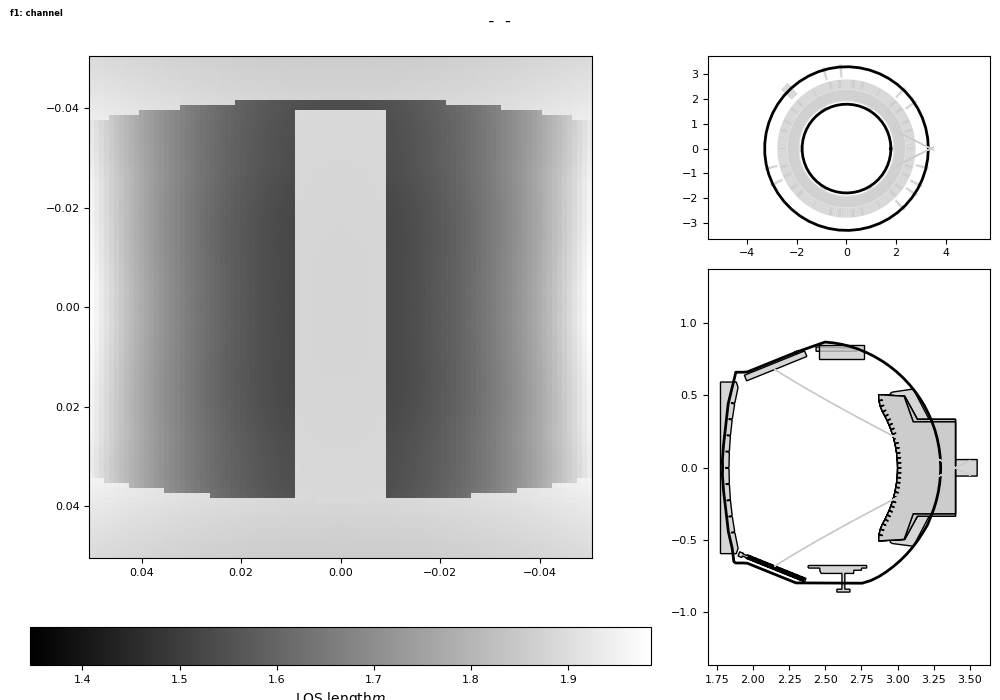# Getting started: 5 minutes tutorial for tofu¶

This is a tutorial that aims to get a new user a little familiar with tofu’s structure.

```# The following imports matplotlib, preferably using a
# backend that allows the plots to be interactive (Qt5Agg).
import numpy as np
import matplotlib
try:
matplotlib.use('Qt5Agg')
except ImportError:
matplotlib.use(matplotlib.rcParams['backend'])
import matplotlib.pyplot
```

We start by loading tofu. You might see some warnings at this stage since optional modules for tofu could be missing on the machine you are working on. This can be ignored safely.

```import tofu as tf
```

We can now create our first configuration. In tofu speak, a configuration is the geometry of the device and its structures. tofu provides pre-defined ones for your to try, so we’re going to do just that:

```configB2 = tf.geom.utils.create_config("B2")
```

The configuration can easily be visualized using the .plot() method:

```configB2.plot()
```Since tofu is all about tomography, let’s create a 1D camera and plot its output.

```cam1d = tf.geom.utils.create_CamLOS1D(
config=configB2,
P=[3.4, 0, 0],
N12=100,
F=0.1,
D12=0.1,
angs=[np.pi, 0, 0],
Name="",
Exp="",
Diag="",
)

# interactive plot
cam1d.plot_touch()
```Out:

```/home/lasofivec/tofu/tofu/utils.py:3230: UserWarning: Not interactive backend!:
- backend : agg   (prefer Qt5Agg)
- canvas  : FigureCanvasAgg
warnings.warn(msg)
```

The principle is similar for 2D cameras.

```cam2d = tf.geom.utils.create_CamLOS2D(
config=configB2,
P=[3.4, 0, 0],
N12=100,
F=0.1,
D12=0.1,
angs=[np.pi, 0, 0],
Name="",
Exp="",
Diag="",
)
cam2d.plot_touch()
```What comes next is up to you! You could now play with the function parameters (change the cameras direction, refinement, aperture), with the plots (many are interactive) or create you own tomographic configuration.

Total running time of the script: ( 0 minutes 2.436 seconds)

Gallery generated by Sphinx-Gallery